# Appendix: Excel Iteration Guide

## How to turn on “Iterations” in Excel

Step 1: After you open a new notebook, click the “File” session in the header. Note that you will only be able to turn on iterations when there is a notebook open.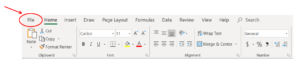Step 2: Select the “Options” appearing at the bottom of the menu.Step 3: Check the “Enable Iterative Calculations” in the “Formulas” session and click “OK”. This will allow excel to perform looped calculations when every variable is dependent on previous variables in the loop, for example, calculating flowrates when a recycle stream is present.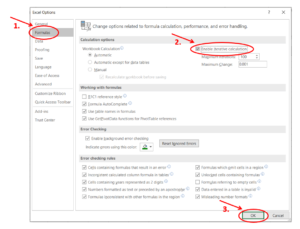Sample Question

Grain extract is produced by the process below. Ethanol is synthesized by yeast in the reactor. In this idealistic process, the yeast converts 2kg of grain into 1 kg of ethanol and 1 kg of water. A perfectly efficient yeast reactor (efficiency = 1) would convert all the grain entering the reactor. A reactor with efficiency =0.5 would convert half of the grain entering the reactor and so on. Below are some additional specifications:

• The feed is 100 kg/min, 20 wt% grain, 80 wt% water
• The reactor efficiency is 0.25
• The grain in the slurry retains 1kg of ethanol-water solution for every 10kg of grain
• 5 wt% of the slurry is purged.
• The mass ratio of water and ethanol in the slurry is the same as in the reactor effluent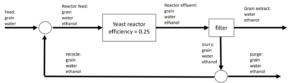## Solution

Step 1: Set up the spreadsheet using the given process specifications and components in each stream as indicated in the question.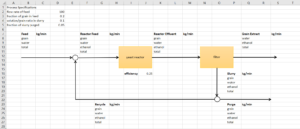Step 2: Express for the individual component flowrate and total flowrate in each stream. For feed:

• total = 100 kg/min (given)
• grain = 0.2*total
• water = total – grain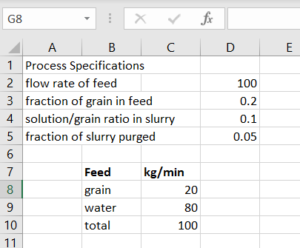Step 3: Reactor Feed:

• grain = grain in feed + grain in recycle
• water = water in feed + water in recycle
• ethanol = ethanol in recycle
• total = grain + water + ethanol

Note: If we reference an empty cell (like the flowrates in the recycle stream), the value will be calculated as 0. This will be updated after we input the formulas for the flow rate in the recycle stream.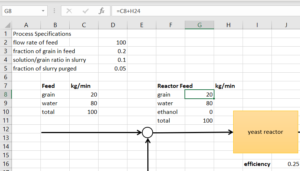Step 4: Reactor effluent:

• grain = grain in reactor feed * (1-reactor efficiency)
• water = water in reactor feed + reactor efficiency * grain in reactor feed * 1/2
• ethanol = ethanol in reactor feed + reactor efficiency * grain in reactor feed * 1/2
• total = grain + water + ethanol

Note: The 1/2 in the flowrate calculations for water and ethanol comes from the mass balance for the conversion of grain. 1/2 is the ratio of $\frac{\text{mass of water produced}}{\text{mass of grain consumed}}$ and  $\frac{\text{mass of ethanol produced}}{\text{mass of grain consumed}}$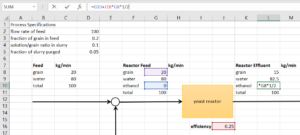Step 5: Slurry:

• grain = grain in reactor effluent
• water = grain in slurry * solution/grain ratio in slurry * $\frac{\text{water in reactor effluent}}{\text{water in reactor effluent+ethanol in reactor effluent}}$
• ethanol = grain in slurry * solution/grain ratio in slurry * $\frac{\text{ethanol in reactor effluent}}{\text{water in reactor effluent+ethanol in reactor effluent}}$
• total = grain + water + ethanol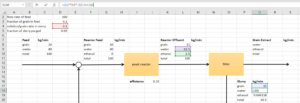Step 6: Grain extract:

• water= water in reactor effluent – water in slurry
• ethanol = ethanol in reactor effluent  – ethanol in slurry
• total = water + ethanol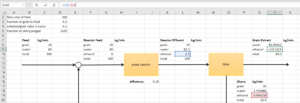Step 7: Purge:

• grain = fraction of slurry purged * grain in slurry
• water = fraction of slurry purged * water in slurry
• ethanol = fraction of slurry purged * ethanol in slurry
• total = grain + water + ethanol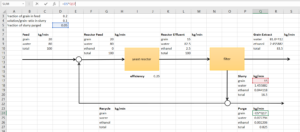Step 8: Recycle:

• grain = grain in slurry – grain in purge
• water = water in slurry – water in purge
• ethanol = ethanol in slurry – ethanol in purge
• total = grain + water + ethanol

Because the recycle stream is the last step in the loop, we can see the other values being updated as we input the formulas for flow rates in the recycle stream.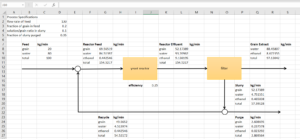Now the flow rates of all streams and all components in each stream are fully solved.